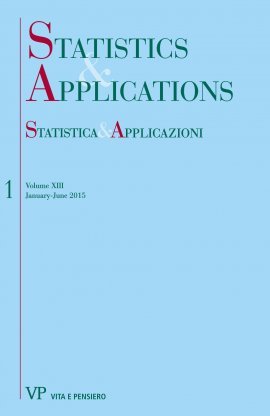Your browser does not support JavaScript!

STATISTICA & APPLICAZIONI - 2015 - 1

digitalDigital issue
 journal STATISTICA & APPLICAZIONI issue 1 - 2015 title STATISTICA & APPLICAZIONI - 2015 - 1 publisher Vita e Pensiero formatDigital issue |Pdf Write a comment for this product

Ebook format Pdf readable on these devices:

• Computer
with Software:
• Android
with App:
• Ibs Tolino
• Kobo
Primo fascicolo del 2015

CONTENTS

A Generalized multivariate skew-normal distribution with applications to spatial and regression predictions
by Z.R. Al-Rawi, Mohd T. Alodat, T.T. Alodat pages: 35
Abstract
In this paper, a generalization to the multivariate skew-normal distribution of Arnold and Beaver (2002) is proposed. Also several distributional properties of the proposed distribution are explored. The proposed distribution has been used to define a stochastic process called the generalized-skew Gaussian process. Furthermore, the paper focuses on applying the proposed distribution to two prediction problems namely, ordinary Kriging and Gaussian process for regression. It is shown that, if the sampling points of the generalized-skew Gaussian processes are chosen so that they are the vertices of a regular polygon, then the ordinary Kriging admit a uniformly best linear unbiased predictor. Finally, we re-analyzed the Gaussian process for regression model under the generalized-skew Gaussian process.
The reordering variates in the decomposition by sources of inequality indexes
by Michele Zenga pages: 38
Abstract
Keywords: Reordering Variate, Income Inequality, Decomposition by Sources, Point Inequality, Uniform Cograduation
On the distribution of the sum of cograduated discrete random variables with applications to credit risk analysis
by Lucio De Capitani, Michele M. Zenga pages: 29
Abstract
This paper focuses on the notion of cograduation which was first introduced in 1939 by the Italian statistician Tommaso Salvemini. In few words, a certain number of random variables are cograduated if they are associated with the maximum positive dependence. Here, it is shown how to derive the probability distribution of the sum of cograduated discrete random variables. This theoretical result is applied to the CreditMetrics and CreditRisk+ models in order to study the probability distribution of the value/loss of a credits portfolio under the assumption of cograduation of single credits. These applications are particularly meaningful since cograduation represents the ‘‘worst scenario’’ which is useful in order to obtain a prudential evaluation of the risk implicit in the credits portfolio.
The distribution of the absolute value of the ratio of two correlated normal random variables
by Angiola Pollastri, Vanda Tulli pages: 13
Abstract
The aim of this paper is to study the distribution of the absolute quotient of two Correlated Normal random variables (r.v.s). This study may have many applications, as often the researcher expects a ratio to be positive or considers the sign of the ratio unimportant. Taking into account a form of the distribution of the ratio of two Correlated Normal r.v.s proposed by Oksoy and Aroian in 1986 and further studied in 1994, we find the distribution of the absolute quotient of two correlated Normal r.v.s. The form proposed here is simple to compute, as it is a function of the Tðh; Þ studied by Owen in 1956. We also represent the distribution of the absolute ratio as a function of the distribution of the Arctangent r.v.. Some examples regarding the confidence intervals for indicators used to evaluate the relative errors of the invoices reveal the importance of the distribution of the absolute ratio of two Correlated Normal r.v.s, here presented.
Assessment of covariance structure in longitudinal analysis
by J. Mohanraj, M.R. Srinivasan pages: 16
Abstract
Linear mixed model with various covariance structures have become increasingly popular in longitudinal data analysis. In this paper estimation of parameters with distinct covariance structures using Maximum Likelihood (ML) and Restricted Maximum Likelihood (REML) methods are considered for evaluation of models using six information criteria, namely AIC, AICC, HQIC, BIC, CAIC and AVIC. The study also involves both the nested and non-nested covariance structure for comparison based on model selection information criteria.